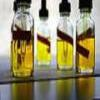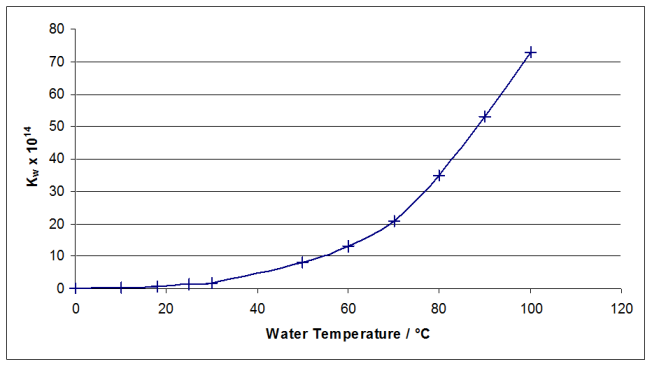#### You may also like### Mathematical Issues for Chemists

A brief outline of the mathematical issues faced by chemistry students.### Reaction Rates

Explore the possibilities for reaction rates versus concentrations with this non-linear differential equation### Catalyse That!

Can you work out how to produce the right amount of chemical in a temperature-dependent reaction?

# pH Temperature

##### Age 16 to 18Challenge Level

The initial key to this problem is to realise that for self-dissociating water, [H]$^+$ = [OH]$^-$.

Therefore, K$_W = [H^+]^2$

Since pH = $-log_{10}[H^+]$

$\mathbf{\Rightarrow K_W = 10^{-2pH}}$

When pH = 7; $K_W = 1 \times 10^{-14}$
pH =6.8; $K_W = 2.51 \times 10^{-14}$
pH = 7.2; $K_W = 0.398 \times 10^{-14}$

Plotting a graph of $K_W$ versus temperature gives:By drawing a smooth curve of best fit through the points, the relevant temperatures can be read off the graph for the given values of $K_W$. If you draw the graph for the entire temperature range, it is difficult to read off accurate values in the required range, which are all smaller than $10\times 10^{14}$. Plotting the curve through the first three points allows us to read off accurate values.